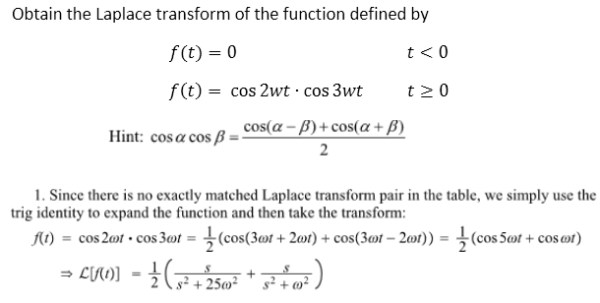Obtain the Laplace transform of the function defined by f(t)=0 for t less than 0 and f(t)=cos(2wt)cos(3wt) for t greater than 0.Obtain the Laplace transform of the function defined by f(t)=0 for t less than 0 and f(t)=cos(2wt)cos(3wt) for t greater than 0.

System Dynamics Page 2 dynamics dynamics dynamics dynamics dynamics dynamics dynamics System dynamics Page 3